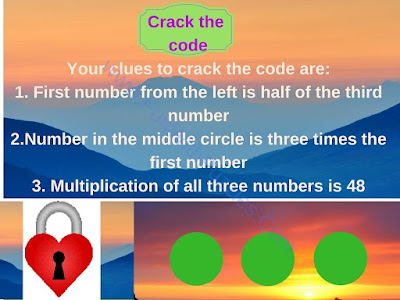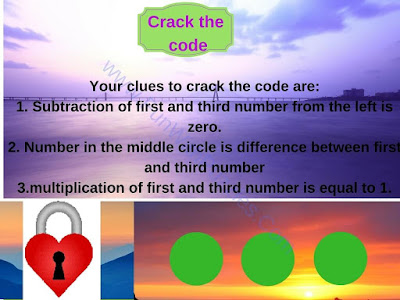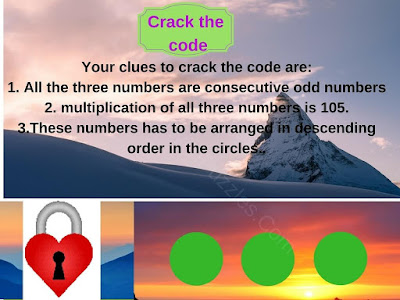My earlier post on Crack the code contained logical puzzles. In those puzzles, one has to find the code which will open the lock and some logical statements were given which will lead to finding the answer to the crack the code problem.
Today's Crack the Code Brain Teasers are Mathematical Puzzles. In these puzzles, few Mathematical statements are given which are hints to solve the lock code. One has to solve these math equations to find the correct answer and thus break the code to open to the lock. Let us see how many locks you can open without looking at the answers.1. Will you crack the code to open the lock?2. Can you crack the code to open the Lock?3. Can you solve this crack the Code Brain Teaser?4. Can you solve this crack the Code puzzle?

Check out some mind crackling brain teasers as mentioned below
List of Brain Teasing Puzzles and Riddles
1. Mathematical Reasoning Puzzles: Do check out these Mathematical Puzzles in which Logical Reasoning along with your Maths skills.

2. Out of Box Thinking Brain Teasers for Students with Answers: These are brain teasers that will make you think out of the box.

3. Out of Box Thinking Fun Brain Teasers with answers to Challenge your Mind: Another set of Out of Box thinking fun brain teasers to challenge your mind.

4. Critical Thinking Puzzles-Crack the Code: These the Critical thinking Crack the Code puzzles. In these puzzles, you will require your logical skill more than your mathematical skill :).

#### 1 comment:

Unknown said...

Find the mistake in Sudoku (1)
Instead of double two's in one column and one row, the common 2 should be changed to 3.
Retired Civil Engineering Professor P. RAJAYOGAN